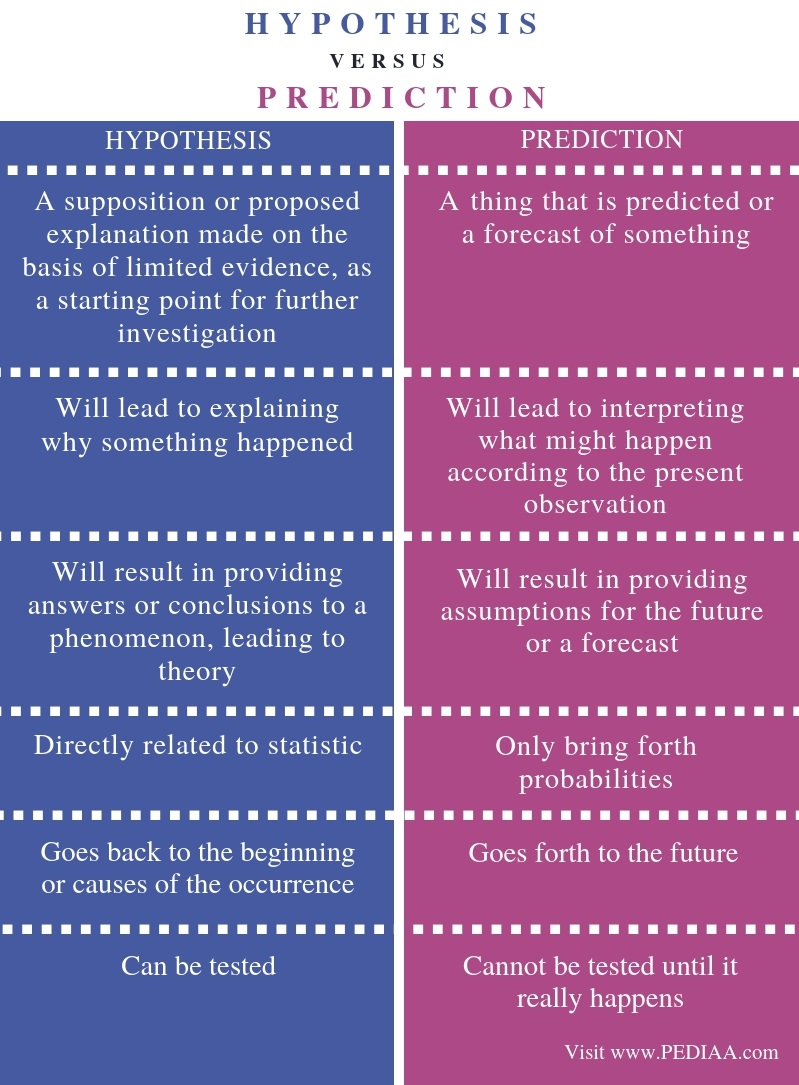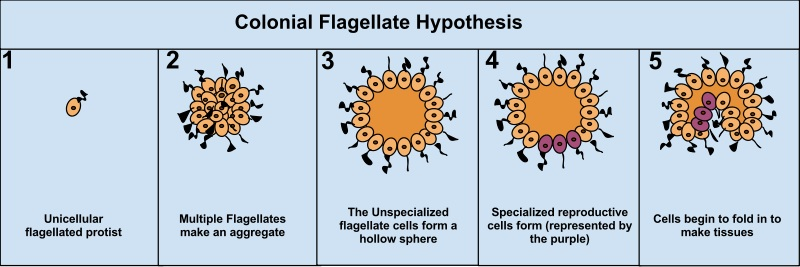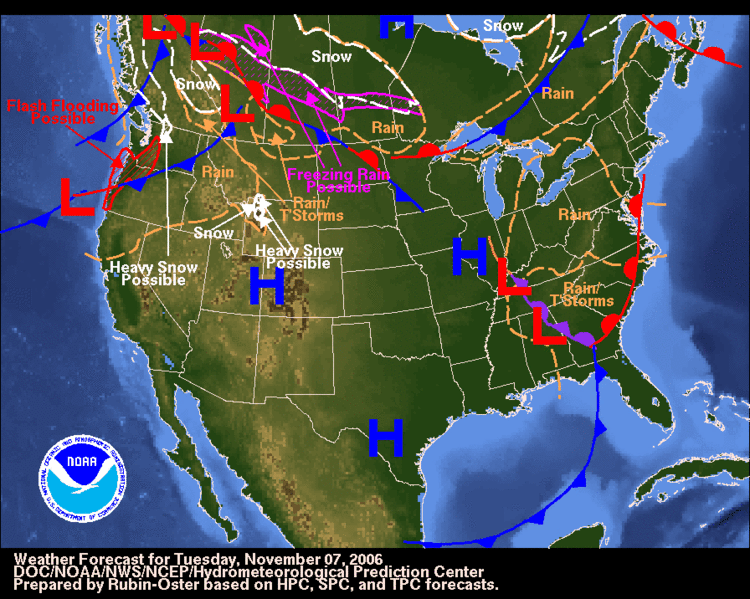# What is the Difference Between Hypothesis and Prediction

The main difference between hypothesis and prediction is that the hypothesis proposes an explanation to something which has already happened whereas the prediction proposes something that might happen in the future.

Hypothesis and prediction are two significant concepts that give possible explanations to several occurrences or phenomena. As a result, one may be able to draw conclusions that assist in formulating new theories, which can affect the future advancements in the human civilizations. Thus, both these terms are common in the field of science, research and logic. In addition, to make a prediction, one should need evidence or observation whereas one can formulate a hypothesis based on limited evidence.

### Key Areas Covered

1. What is a Hypothesis
– Definition, Features
2. What is a Prediction
– Definition, Features
3. What is the Relationship Between Hypothesis and Prediction
– Outline of Common Features
4. What is the Difference Between Hypothesis and Prediction
– Comparison of Key Differences

### Key Terms

Hypothesis, Logic, Prediction, Theories, Science## What is a Hypothesis

By definition, a hypothesis refers to a supposition or a proposed explanation made on the basis of limited evidence as a starting point for further investigation. In brief, a hypothesis is a proposed explanation for a phenomenon.  Nevertheless, this is based on limited evidence, facts or information one has based on the underlying causes of the problem. However, it can be further tested by experimentation. Therefore, this is yet to be proven as correct.

This term hypothesis is, thus, used often in the field of science and research than in general usage. In science, it is termed as a scientific hypothesis. However, a scientific hypothesis has to be tested by a scientific method. Moreover, scientists usually base scientific hypotheses on previous observations which cannot be explained by the existing scientific theories.Figure 01: A Hypothesis on Colonial Flagellate

In research studies, a hypothesis is based on independent and dependent variables. This is known as a ‘working hypothesis’, and it is provisionally accepted as a basis for further research, and often serves as a conceptual framework in qualitative research. As a result, based on the gathered facts in research, the hypothesis tends to create links or connections between the different variables. Thus, it will work as a source for a more concrete scientific explanation.

Hence, one can formulate a theory based on the hypothesis to lead on the investigation to the problem. A strong hypothesis can create effective predictions based on reasoning. As a result, a hypothesis can predict the outcome of an experiment in a laboratory or the observation of a natural phenomenon. Hence, a hypothesis is known as an ‘educated guess’.

## What is a Prediction

A prediction can be defined as a thing predicted or a forecast. Hence, a prediction is a statement about something that might happen in the future. Thus, one can guess as to what might happen based on the existing evidence or observations.

In the general context, although it is difficult to predict the uncertain future, one can draw conclusions as to what might happen in the future based on the observations of the present. This will assist in avoiding negative consequences in the future when there are dangerous occurrences in the present.

Moreover, there is a link between hypothesis and prediction. A strong hypothesis will enable possible predictions. This link between a hypothesis and a prediction can be clearly observed in the field of science.Figure 2: Weather Predictions

Hence, in scientific and research studies, a prediction is a specific design that can be used to test one’s hypothesis. Thus, the prediction is the outcome one can observe if their hypothesis were supported with experiment. Moreover, predictions are often written in the form of “if, then” statements; for example, “if my hypothesis is true, then this is what I will observe.”

## Relationship Between Hypothesis and Prediction

• Based on a hypothesis, one can create a prediction
• Also, a hypothesis will enable predictions through the act of deductive reasoning.
• Furthermore, the prediction is the outcome that can be observed if the hypothesis were supported proven by the experiment.

## Difference Between Hypothesis and Prediction

### Definition

Hypothesis refers to the supposition or proposed explanation made on the basis of limited evidence, as a starting point for further investigation. On the other hand, prediction refers to a thing that is predicted or a forecast of something. Thus, this explains the main difference between hypothesis and prediction.

### Interpretation

Hypothesis will lead to explaining why something happened while prediction will lead to interpreting what might happen according to the present observations. This is a major difference between hypothesis and prediction.

### Result

Another difference between hypothesis and prediction is that hypothesis will result in providing answers or conclusions to a phenomenon, leading to theory, while prediction will result in providing assumptions for the future or a forecast.

### Statistics

While a hypothesis is directly related to statistics, a prediction, though it may invoke statistics, will only bring forth probabilities.

### Process

Moreover, hypothesis goes back to the beginning or causes of the occurrence while prediction goes forth to the future occurrence.

### Nature

The ability to be tested is another difference between hypothesis and prediction. A hypothesis can be tested, or it is testable whereas a prediction cannot be tested until it really happens.

### Conclusion

Hypothesis and prediction are integral components in scientific and research studies. However, they are also used in the general context. Hence, hypothesis and prediction are two distinct concepts although they are related to each other as well. The main difference between hypothesis and prediction is that hypothesis proposes an explanation to something which has already happened whereas prediction proposes something that might happen in the future.

##### Reference:

1. “Prediction.” Wikipedia, Wikimedia Foundation, 17 Sept. 2018, Available here.
2. “Hypothesis.” Wikipedia, Wikimedia Foundation, 20 Sept. 2018, Available here.
3. Bradford, Alina. “What Is a Scientific Hypothesis? | Definition of Hypothesis.” LiveScience, Purch, 26 July 2017, Available here.
4. “Understanding Hypotheses and Predictions.” The Academic Skills Centre Trent University, Available here.

##### Image Courtesy:

1. “Colonial Flagellate Hypothesis” By Katelynp1 – Own work (CC BY-SA 3.0) via Commons Wikimedia
2. “USA weather forecast 2006-11-07” By NOAA – (Public Domain) via Commons Wikimedia